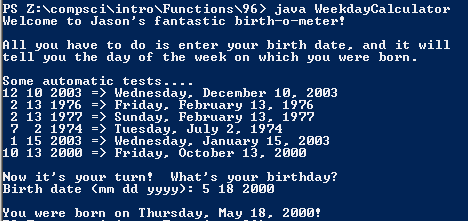# Assignment #96 and WeekdayCalculator

## Code

```      /// Name: Jason Kim
/// Period: 7
/// Program Name: WeekdayCalculator
/// File Name: WeekdayCalculator.java
/// Date Finished:1/21/16

import java.util.Scanner;

public class WeekdayCalculator
{
public static void main( String[] args )
{
Scanner keyboard = new Scanner(System.in);

System.out.println("Welcome to Jason's fantastic birth-o-meter!");
System.out.println();
System.out.println("All you have to do is enter your birth date, and it will");
System.out.println("tell you the day of the week on which you were born.");
System.out.println();
System.out.println("Some automatic tests....");
System.out.println("12 10 2003 => " + weekday(12,10,2003));
System.out.println(" 2 13 1976 => " + weekday(2,13,1976));
System.out.println(" 2 13 1977 => " + weekday(2,13,1977));
System.out.println(" 7  2 1974 => " + weekday(7,2,1974));
System.out.println(" 1 15 2003 => " + weekday(1,15,2003));
System.out.println("10 13 2000 => " + weekday(10,13,2000));
System.out.println();

System.out.print("Birth date (mm dd yyyy): ");
int mm = keyboard.nextInt();
int dd = keyboard.nextInt();
int yyyy = keyboard.nextInt();
System.out.println("\nYou were born on " + weekday(mm, dd, yyyy) + "!");
}

public static String weekday( int mm, int dd, int yyyy )
{
int yy = yyyy - 1900, total;
String date = "";

total = (yy - yy%4)/4 + yy + dd + monthOffset(mm);
if ( isLeap(yy) && (mm == 1 || mm == 2))
{
total = total - 1;
}
int remainder = total%7;
String dayOfWeek = weekday_name (remainder);

date = dayOfWeek + ", " +  month_name(mm) + " " + dd +", " + yyyy;

return date;
}

public static int monthOffset( int month )
{
int result;
if ( month == 1 )
{
result = 1;
}
else if ( month == 2 )
{
result = 4;
}
else if ( month == 3 )
{
result = 4;
}
else if ( month == 4 )
{
result = 0;
}
else if ( month == 5)
{
result = 2;
}
else if ( month == 6)
{
result = 5;
}
else if ( month == 7)
{
result = 0;
}
else if ( month == 8)
{
result = 3;
}
else if ( month == 9)
{
result = 6;
}
else if ( month == 10)
{
result = 1;
}
else if ( month == 11)
{
result = 4;
}
else if ( month == 12)
{
result = 6;
}
else
{
result = -1;
}

return result;
}

public static String month_name( int month )
{
String result;
if ( month == 1)
{
result = "January";
}
else if ( month == 2)
{
result = "February";
}
else if (month == 3)
{
result = "March";
}
else if (month == 4)
{
result = "April";
}
else if (month == 5)
{
result = "May";
}
else if (month == 6)
{
result = "June";
}
else if (month == 7)
{
result = "July";
}
else if (month == 8)
{
result = "August";
}
else if (month == 9)
{
result = "September";
}
else if (month == 10)
{
result = "October";
}
else if (month == 11)
{
result = "November";
}
else if (month == 12)
{
result = "December";
}
else
{
result = "error";
}
return result;
}
public static String weekday_name( int weekday )
{
String result = "";

if ( weekday == 1 )
{
result = "Sunday";
}
else if ( weekday == 2 )
{
result = "Monday";
}
else if ( weekday == 3 )
{
result = "Tuesday" ;
}
else if ( weekday == 4 )
{
result = "Wednesday" ;
}
else if ( weekday == 5 )
{
result = "Thursday" ;
}
else if ( weekday == 6 )
{
result = "Friday" ;
}
else if ( weekday == 7 )
{
result = "Saturday";
}
else if ( weekday == 0 )
{
result = "Saturday";
}
else
{
result = "error" ;
}
return result;
}
public static boolean isLeap( int year )
{
boolean result;

if ( year%400 == 0 )
result = true;
else if ( year%100 == 0 )
result = false;
else if ( year%4 == 0 )
result = true;
else
result = false;

return result;
}
}
```

### Picture of the output